# Should you keep the first Envelope or change? Choice between “Two Envelopes Paradox”

## Related Post

You are offered a choice between two envelopes E1 and E2. All that you know about them is that one contains twice as much money as the other. After you have chosen one of the envelopes, you are offered the opportunity to switch the envelope that you have chosen for the other envelope.

Should you keep the first Envelope or change?

Here is a tempting line of reasoning that suggests that you should make this trade. Suppose that you have chosen E1. The amount of money in E1 is $x. Then the amount of money in E2 is either$2x or $0.5x Moreover—since your choice between the two envelopes was undertaken in complete ignorance of the contents of the two envelopes—you should regard the two possible outcomes as equally likely. 0.5×$2x+0.5×$0.5x=$1.25x

Since the expected utility of trading E1 for E2 is greater than the expected utility of hanging on to E1, you should switch. (1.25 is greater than 1.0 … RIGHT????)

you should switch
. 1.25 is greater than 1.0 … RIGHT????

To find out write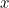for the amount that’s in your chosen envelope. This means that the amount of money in the other envelope is either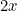or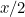. The probability that it’sis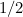and so is the probability that it’s. So the expected amount you’ll get for switching is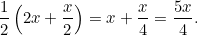Since that’s bigger than, you should swap envelopes. But what if you are given another chance to swap envelopes after you have changed your mind once? By the same reasoning as above you should swap back again. And then, by the same argument again, you should swap a third time, and so on, forever. You end up in an infinite loop of swapping and never get any money at all. What’s wrong with this reasoning?

### A resolution

Let’s write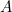for the envelope you picked at first and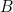for the other one. We writefor the amount of money in. Now since we haven’t opened envelope,isn’t a fixed amount: it’s a random variable. It can take one of two values: the smaller amount of money that’s hidden in the two envelopes or the larger amount of money. Let’s write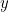for the smaller amount and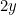for the larger amount (recall that one envelope contains twice as much money as the other). Since you have pickedrandomly, there’s a 50:50 chance thatcontains either of the two amounts. This means that the expected amount of money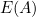in envelopeis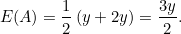We said above that the expected amount in envelopeis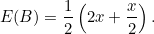(1)

But recall thatisn’t a fixed amount but can take one of two values. In the case that envelopecontains, envelopecontains the smaller amount of money, so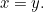In the case that envelopecontains, envelopecontains the larger amount of money, so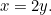So in formula (1) above, the firstreally stands forand the secondstands for. The two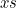in the formula are actually different and shouldn’t have been added up to give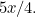Substituting thefor the first appearance ofin (1) andfor the second gives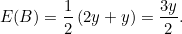Thus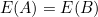so there is no incentive to switch envelopes and hence no paradox.

VN:F [1.9.22_1171]